# 4.1 Linear functions  (Page 9/27)

 Page 9 / 27

## Vertical stretch or compression

In the equation $\text{\hspace{0.17em}}f\left(x\right)=mx,$ the $\text{\hspace{0.17em}}m\text{\hspace{0.17em}}$ is acting as the vertical stretch    or compression of the identity function. When $\text{\hspace{0.17em}}m\text{\hspace{0.17em}}$ is negative, there is also a vertical reflection of the graph. Notice in [link] that multiplying the equation of $\text{\hspace{0.17em}}f\left(x\right)=x\text{\hspace{0.17em}}$ by $\text{\hspace{0.17em}}m\text{\hspace{0.17em}}$ stretches the graph of $\text{\hspace{0.17em}}f\text{\hspace{0.17em}}$ by a factor of $\text{\hspace{0.17em}}m\text{\hspace{0.17em}}$ units if $\text{\hspace{0.17em}}m>\text{1}\text{\hspace{0.17em}}$ and compresses the graph of $\text{\hspace{0.17em}}f\text{\hspace{0.17em}}$ by a factor of $\text{\hspace{0.17em}}m\text{\hspace{0.17em}}$ units if $\text{\hspace{0.17em}}0 This means the larger the absolute value of $\text{\hspace{0.17em}}m,\text{\hspace{0.17em}}$ the steeper the slope.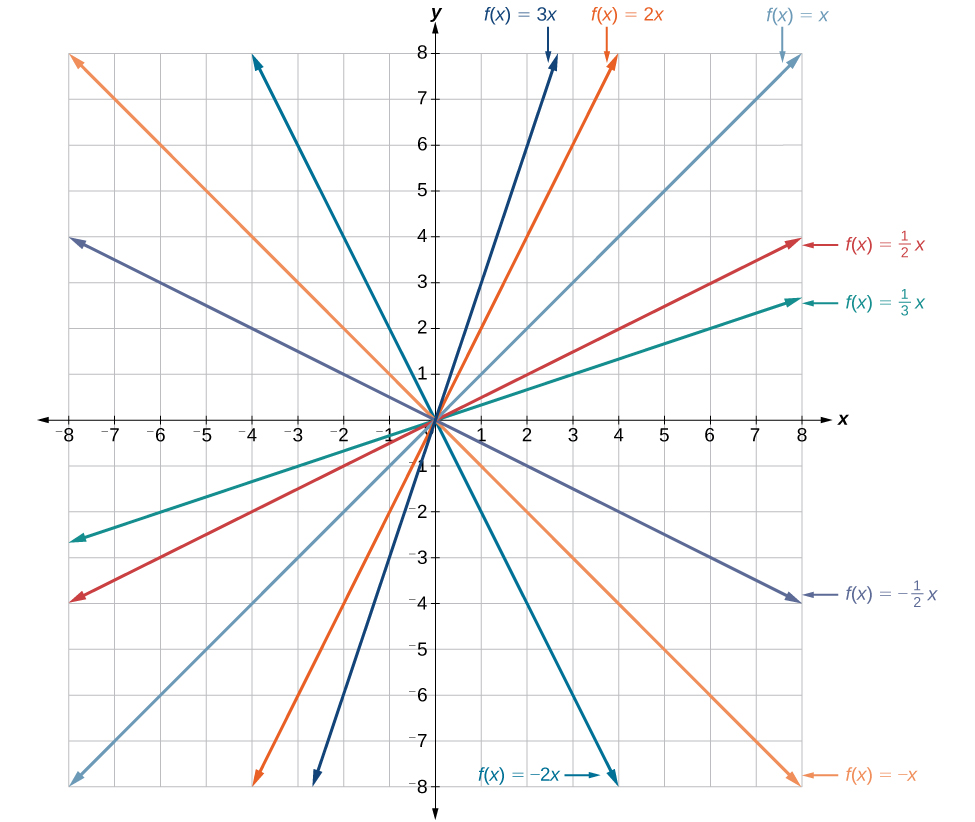Vertical stretches and compressions and reflections on the function   f ( x ) = x

## Vertical shift

In $\text{\hspace{0.17em}}f\left(x\right)=mx+b,$ the $\text{\hspace{0.17em}}b\text{\hspace{0.17em}}$ acts as the vertical shift    , moving the graph up and down without affecting the slope of the line. Notice in [link] that adding a value of $\text{\hspace{0.17em}}b\text{\hspace{0.17em}}$ to the equation of $\text{\hspace{0.17em}}f\left(x\right)=x\text{\hspace{0.17em}}$ shifts the graph of $\text{\hspace{0.17em}}f\text{\hspace{0.17em}}$ a total of $\text{\hspace{0.17em}}b\text{\hspace{0.17em}}$ units up if $\text{\hspace{0.17em}}b\text{\hspace{0.17em}}$ is positive and $\text{\hspace{0.17em}}|b|\text{\hspace{0.17em}}$ units down if $\text{\hspace{0.17em}}b\text{\hspace{0.17em}}$ is negative.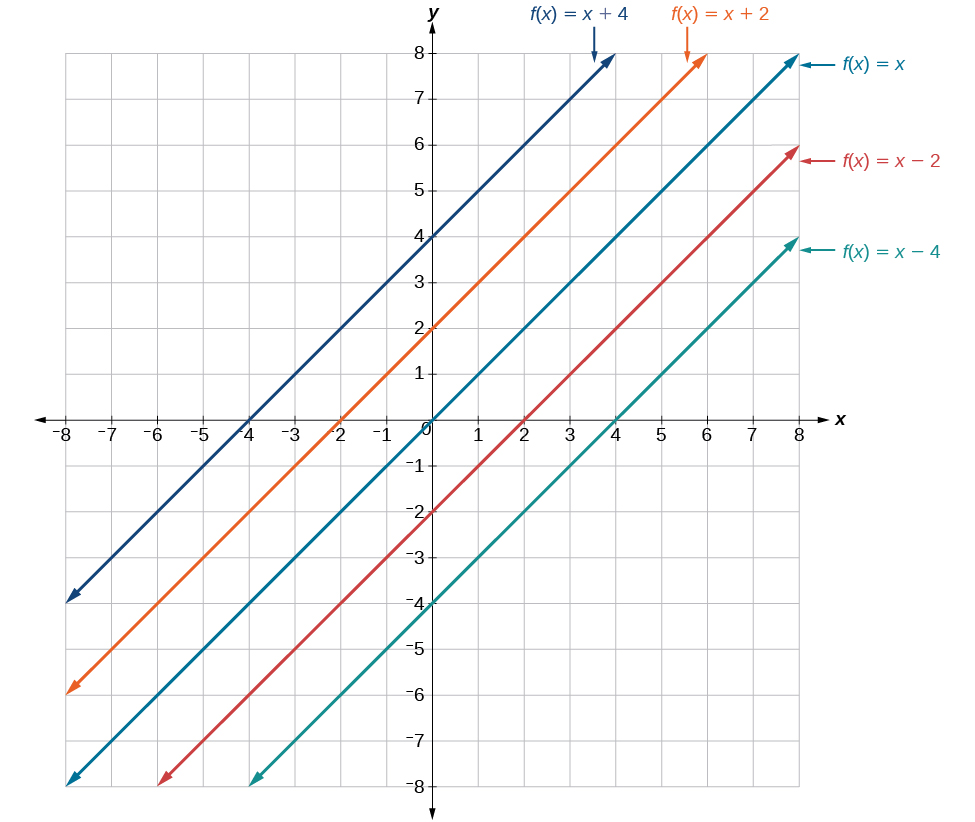This graph illustrates vertical shifts of the function f ( x ) = x .

Using vertical stretches or compressions along with vertical shifts is another way to look at identifying different types of linear functions. Although this may not be the easiest way to graph this type of function, it is still important to practice each method.

Given the equation of a linear function, use transformations to graph the linear function in the form $\text{\hspace{0.17em}}f\left(x\right)=mx+b.$

1. Graph $\text{\hspace{0.17em}}f\left(x\right)=x.$
2. Vertically stretch or compress the graph by a factor $\text{\hspace{0.17em}}m.$
3. Shift the graph up or down $\text{\hspace{0.17em}}b\text{\hspace{0.17em}}$ units.

## Graphing by using transformations

Graph $\text{\hspace{0.17em}}f\left(x\right)=\frac{1}{2}x-3\text{\hspace{0.17em}}$ using transformations.

The equation for the function shows that $\text{\hspace{0.17em}}m=\frac{1}{2}\text{\hspace{0.17em}}$ so the identity function is vertically compressed by $\text{\hspace{0.17em}}\frac{1}{2}.\text{\hspace{0.17em}}$ The equation for the function also shows that $\text{\hspace{0.17em}}b=-3\text{\hspace{0.17em}}$ so the identity function is vertically shifted down 3 units. First, graph the identity function, and show the vertical compression as in [link] .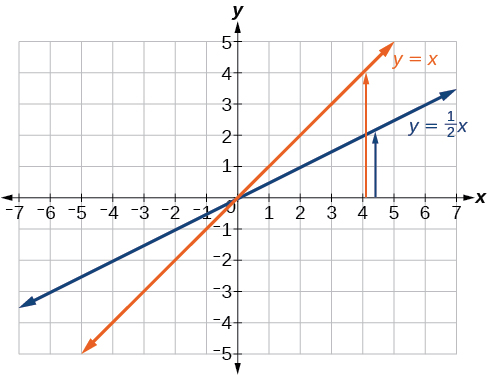The function,   y = x , compressed by a factor of   1 2

Then show the vertical shift as in [link] .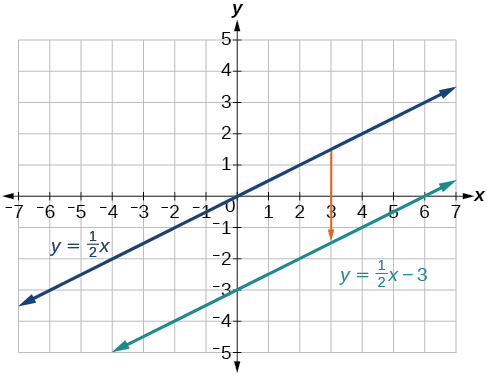The function   y = 1 2 x , shifted down 3 units

Graph $\text{\hspace{0.17em}}f\left(x\right)=4+2x\text{\hspace{0.17em}}$ using transformations.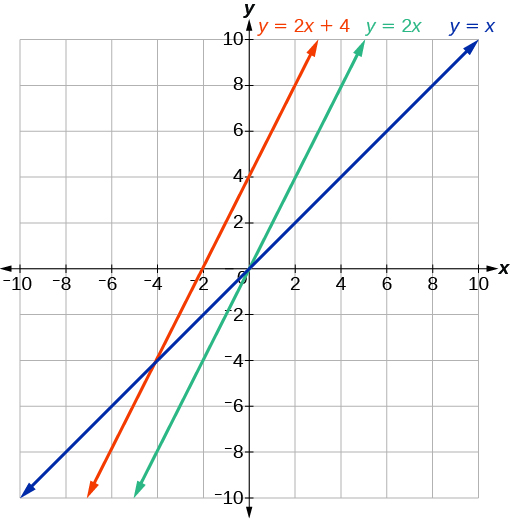In [link] , could we have sketched the graph by reversing the order of the transformations?

No. The order of the transformations follows the order of operations. When the function is evaluated at a given input, the corresponding output is calculated by following the order of operations. This is why we performed the compression first. For example, following the order: Let the input be 2.

$\begin{array}{ccc}\hfill f\left(2\right)& =& \frac{1}{2}\left(2\right)-3\hfill \\ & =& 1-3\hfill \\ & =& -2\hfill \end{array}$

## Writing the equation for a function from the graph of a line

Earlier, we wrote the equation for a linear function from a graph. Now we can extend what we know about graphing linear functions to analyze graphs a little more closely. Begin by taking a look at [link] . We can see right away that the graph crosses the y -axis at the point $\text{\hspace{0.17em}}\left(0,\text{4}\right)\text{\hspace{0.17em}}$ so this is the y -intercept.

Then we can calculate the slope by finding the rise and run. We can choose any two points, but let’s look at the point $\text{\hspace{0.17em}}\left(–2,0\right).\text{\hspace{0.17em}}$ To get from this point to the y- intercept, we must move up 4 units (rise) and to the right 2 units (run). So the slope must be

$m=\frac{\text{rise}}{\text{run}}=\frac{4}{2}=2$

Substituting the slope and y- intercept into the slope-intercept form of a line gives

#### Questions & Answers

if sin15°=√p, express the following in terms of p
Norman Reply
prove sin²x+cos²x=3+cos4x
Kiddy Reply
the difference between two signed numbers is -8.if the minued is 5,what is the subtrahend
jeramie Reply
the difference between two signed numbers is -8.if the minuend is 5.what is the subtrahend
jeramie
what are odd numbers
micheal Reply
numbers that leave a remainder when divided by 2
Thorben
1,3,5,7,... 99,...867
Thorben
7%2=1, 679%2=1, 866245%2=1
Thorben
the third and the seventh terms of a G.P are 81 and 16, find the first and fifth terms.
Suleiman Reply
if a=3, b =4 and c=5 find the six trigonometric value sin
Martin Reply
ask
Ans
pls how do I factorize x⁴+x³-7x²-x+6=0
Gift Reply
in a function the input value is called
Rimsha Reply
how do I test for values on the number line
Modesta Reply
if a=4 b=4 then a+b=
Rimsha Reply
a+b+2ab
Kin
commulative principle
DIOSDADO
a+b= 4+4=8
Mimi
If a=4 and b=4 then we add the value of a and b i.e a+b=4+4=8.
Tariq
what are examples of natural number
sani Reply
an equation for the line that goes through the point (-1,12) and has a slope of 2,3
Katheryn Reply
3y=-9x+25
Ishaq
show that the set of natural numberdoes not from agroup with addition or multiplication butit forms aseni group with respect toaaddition as well as multiplication
Komal Reply
x^20+x^15+x^10+x^5/x^2+1
Urmila Reply
evaluate each algebraic expression. 2x+×_2 if ×=5
Sarch Reply

### Read also:

#### Get Jobilize Job Search Mobile App in your pocket Now!

Source:  OpenStax, Algebra and trigonometry. OpenStax CNX. Nov 14, 2016 Download for free at https://legacy.cnx.org/content/col11758/1.6
Google Play and the Google Play logo are trademarks of Google Inc.

Notification Switch

Would you like to follow the 'Algebra and trigonometry' conversation and receive update notifications?ByByBy OpenStaxBy Nicole BartelsBy Michael NelsonBy JavaChamp TeamBy Olivia D'AmbrogioBy Katie MontroseBy Joanna SmithbackBy Brooke DelaneyBy Carly AllenBy David Martin